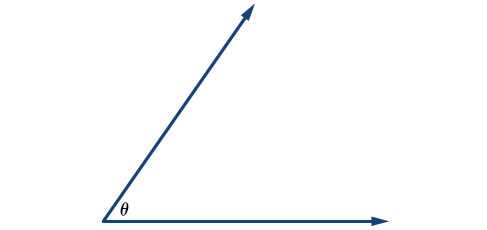# 7.1 Angles

 Page 1 / 29
In this section you will:
• Draw angles in standard position.
• Convert between degrees and radians.
• Find coterminal angles.
• Find the length of a circular arc.
• Use linear and angular speed to describe motion on a circular path.

A golfer swings to hit a ball over a sand trap and onto the green. An airline pilot maneuvers a plane toward a narrow runway. A dress designer creates the latest fashion. What do they all have in common? They all work with angles, and so do all of us at one time or another. Sometimes we need to measure angles exactly with instruments. Other times we estimate them or judge them by eye. Either way, the proper angle can make the difference between success and failure in many undertakings. In this section, we will examine properties of angles.

## Drawing angles in standard position

Properly defining an angle first requires that we define a ray. A ray    is a directed line segment. It consists of one point on a line and all points extending in one direction from that point. The first point is called the endpoint of the ray. We can refer to a specific ray by stating its endpoint and any other point on it. The ray in [link] can be named as ray EF, or in symbol form $\text{\hspace{0.17em}}\stackrel{⟶}{EF}.$

An angle    is the union of two rays having a common endpoint. The endpoint is called the vertex    of the angle, and the two rays are the sides of the angle. The angle in [link] is formed from $\text{\hspace{0.17em}}\stackrel{⟶}{ED}\text{\hspace{0.17em}}$ and $\text{\hspace{0.17em}}\stackrel{⟶}{EF}\text{\hspace{0.17em}}$ . Angles can be named using a point on each ray and the vertex, such as angle DEF , or in symbol form $\text{\hspace{0.17em}}\angle DEF.$

Greek letters are often used as variables for the measure of an angle. [link] is a list of Greek letters commonly used to represent angles, and a sample angle is shown in [link] .

$\theta$ $\phi \text{\hspace{0.17em}}$ or $\text{\hspace{0.17em}}\varphi$ $\alpha$ $\beta$ $\gamma$
theta phi alpha beta gammaAngle theta, shown as   ∠ θ

Angle creation is a dynamic process. We start with two rays lying on top of one another. We leave one fixed in place, and rotate the other. The fixed ray is the initial side     , and the rotated ray is the terminal side    . In order to identify the different sides, we indicate the rotation with a small arrow close to the vertex as in [link] .

As we discussed at the beginning of the section, there are many applications for angles, but in order to use them correctly, we must be able to measure them. The measure of an angle    is the amount of rotation from the initial side to the terminal side. Probably the most familiar unit of angle measurement is the degree. One degree    is $\text{\hspace{0.17em}}\frac{1}{360}\text{\hspace{0.17em}}$ of a circular rotation, so a complete circular rotation contains $\text{\hspace{0.17em}}360\text{\hspace{0.17em}}$ degrees. An angle measured in degrees should always include the unit “degrees” after the number, or include the degree symbol $°.\text{\hspace{0.17em}}$ For example,

To formalize our work, we will begin by drawing angles on an x - y coordinate plane. Angles can occur in any position on the coordinate plane, but for the purpose of comparison, the convention is to illustrate them in the same position whenever possible. An angle is in standard position    if its vertex is located at the origin, and its initial side extends along the positive x -axis. See [link] .

If the angle is measured in a counterclockwise direction from the initial side to the terminal side, the angle is said to be a positive angle    . If the angle is measured in a clockwise direction, the angle is said to be a negative angle    .

#### Questions & Answers

write down the polynomial function with root 1/3,2,-3 with solution
if A and B are subspaces of V prove that (A+B)/B=A/(A-B)
write down the value of each of the following in surd form a)cos(-65°) b)sin(-180°)c)tan(225°)d)tan(135°)
Prove that (sinA/1-cosA - 1-cosA/sinA) (cosA/1-sinA - 1-sinA/cosA) = 4
what is the answer to dividing negative index
In a triangle ABC prove that. (b+c)cosA+(c+a)cosB+(a+b)cisC=a+b+c.
give me the waec 2019 questions
the polar co-ordinate of the point (-1, -1)
prove the identites sin x ( 1+ tan x )+ cos x ( 1+ cot x )= sec x + cosec x
tanh`(x-iy) =A+iB, find A and B
B=Ai-itan(hx-hiy)
Rukmini
what is the addition of 101011 with 101010
If those numbers are binary, it's 1010101. If they are base 10, it's 202021.
Jack
extra power 4 minus 5 x cube + 7 x square minus 5 x + 1 equal to zero
the gradient function of a curve is 2x+4 and the curve passes through point (1,4) find the equation of the curve
1+cos²A/cos²A=2cosec²A-1
test for convergence the series 1+x/2+2!/9x3ByByBy